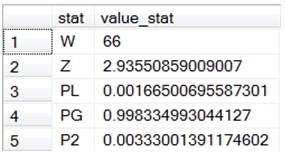# SQL Server Wilcoxon matched-pair signed rank test

WMPSR_TV

Updated: 24 May 2013

Use the table-valued function WMPSR_TV to calculate the Wilcoxon matched-pair signed-rank test.
Syntax
SELECT * FROM [wctStatistics].[wct].[WMPSR_TV](
<@x_y_Query, nvarchar(max),>)
Arguments
@InputData_RangeQuery
a T-SQL statement, as a string, that specifies the matched pairs passed into the function.
Return Type
RETURNS TABLE (
[W] [float] NULL,
[Z] [float] NULL,
[PL] [float] NULL,
[PG] [float] NULL,
[P2] [float] NULL
)

 Column Column Description W the test statistic Z the z test statistic (used in the normal approximation of the p-value) PL the ‘less than’ p-value PG the ‘greater than’ p-value P2 the 2-sided p-value
Remarks
·         The function is insensitive to order; it does not matter what order the pairs are passed in.
·         Ranks are calculated based on the absolute value in the difference of a pair. If we call the first value in the pair and the second y, then the rank is calculated based on ABS(x-y)
·         Pairs where x = y are not included in the rank calculation.
·         Ranks ties are calculated as the average of the rank in the interval occupied by the tie.
·         Once the ranks are calculated, the rank is multiplied by SIGN(x-y). The W-statistic is the sum of absolute values where SIGN(x-y) = 1.
·         If the number of ranked pairs is less than 50, then the exact p-value is calculated using the PSIGNRANK function, otherwise the NORMSDIST function is used.
Examples
SELECT x, y
INTO #w
FROM (
SELECT 5260,3910 UNION ALL
SELECT 5470,4220 UNION ALL
SELECT 5640,3885 UNION ALL
SELECT 6180,5160 UNION ALL
SELECT 6390,5645 UNION ALL
SELECT 6515,4680 UNION ALL
SELECT 6805,5265 UNION ALL
SELECT 7515,5975 UNION ALL
SELECT 7515,6790 UNION ALL
SELECT 8230,6900 UNION ALL
SELECT 8770,7335
) w(x,y)

SELECT p.*
FROM wct.WMPSR_TV('SELECT x,y FROM #w')
--This CROSS APPLY UNPIVOTS the tvf columns for formatting
CROSS APPLY(
SELECT 'W', W UNION ALL
SELECT 'Z', Z UNION ALL
SELECT 'PL', PL UNION ALL
SELECT 'PG', PG UNION ALL
SELECT 'P2', P2
) p(stat, value_stat)
This produces the following result.### SupportCopyright 2008-2021 Westclintech LLC         Privacy Policy        Terms of Service